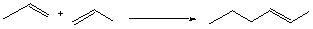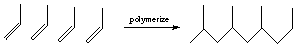Polymers Solutions: #4

4.* (1996 3 2) Consider the thermodynamic factors underlying the polymerization of a simple olefin: propylene.A. Estimate the value of the enthalpy change DH for this polymerization. The bond energies of a carbon-carbon sigma bond and a carbon-carbon pi bond are approximately 86 and 63 Kcal per mole respectively.

For each monomer entering the polymer:

break one p bond: DH = +63 kcal/mol

form one p bond: DH = -86 kcal/mol

DH = -23 kcal/mol

B. Deduce the sign of the enthalpy change for the polymerization.

The sign of the DH term is negative, so the reaction is enthalpically favorable and exothermic.

C. Then deduce the sign of the entropy change for the reaction.

DS, the change in entropy, is negative because we’re putting all the monomers together to make a polymer, which is more ordered than free molecules.

D. What is the sign of the Gibbs free energy change for this reaction? Explain your reasoning and show your calculation.

DG =D H -TDS = -23 kcal/mol - (298)(something negative)

DG will be negative as long as the TDS term is less than 23 kcal/mol (i.e. DG is negative except at high temperature.)

We know that polypropylene is formed from propylene, so DG must be negative under relatively ordinary conditions, so |DH| > |TDS|